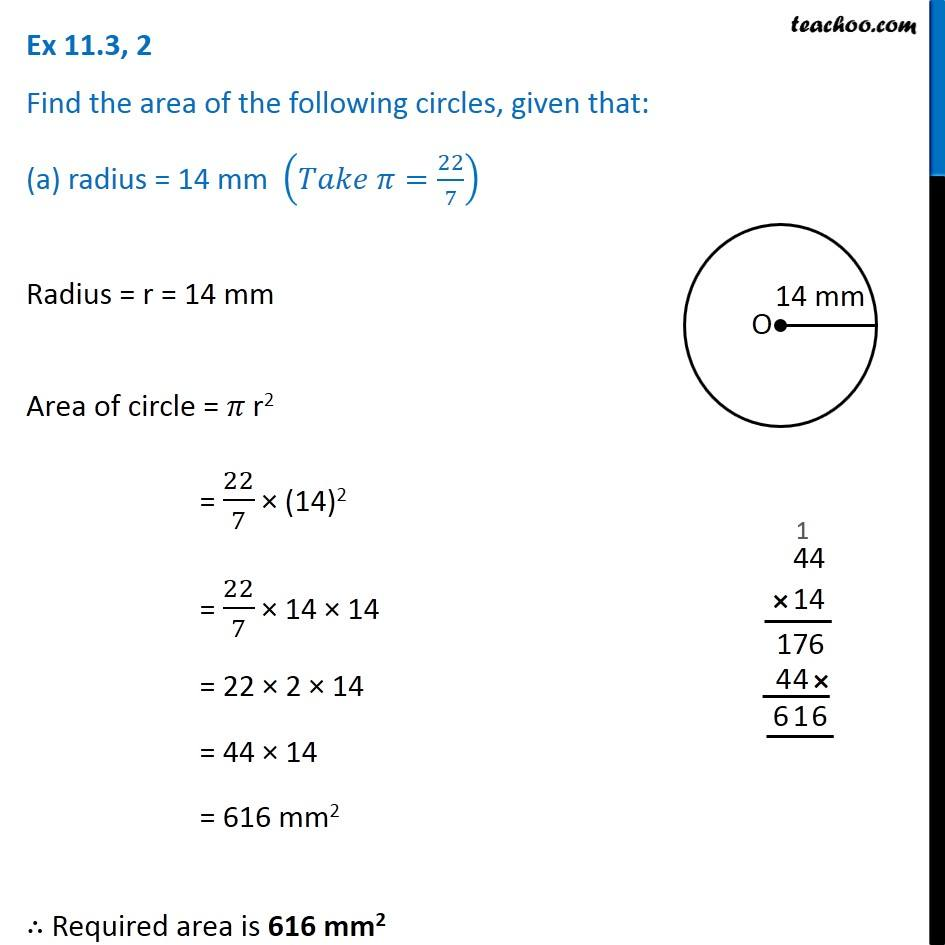Area of circle

Chapter 9 Class 7 Perimeter and Area
Concept wiseLearn in your speed, with individual attention - Teachoo Maths 1-on-1 Class

### Transcript

Ex 9.2, 2 Find the area of the following circles, given that: (a) radius = 14 mm (𝑇𝑎𝑘𝑒 𝜋=22/7) Radius = r = 14 mm Area of circle = 𝜋 r2 = 22/7 × (14)2 = 22/7 × 14 × 14 = 22 × 2 × 14 = 44 × 14 = 616 mm2 ∴ Required area is 616 mm2